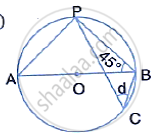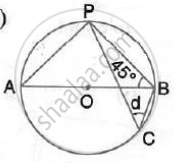Share

Books Shortlist

# In the Following Figures, O is the Centre of the Circle. Find the Values of A, B, C and D. - ICSE Class 10 - Mathematics

ConceptChord Properties - Chords Equidistant from the Center Are Equal (Without Proof)

#### Question

In the following figures, O is the centre of the circle. Find the values of a, b, c and d.#### Solution∠APB = 90°  (Angle in a semicircle)
∴ ∠BAP = 90° - 45° = 45°
Now, d = ∠BCP = ∠BAP = 45°
(Angle subtended by the same chord on the circle are equal)

Is there an error in this question or solution?

#### Video TutorialsVIEW ALL 

Solution In the Following Figures, O is the Centre of the Circle. Find the Values of A, B, C and D. Concept: Chord Properties - Chords Equidistant from the Center Are Equal (Without Proof).
S